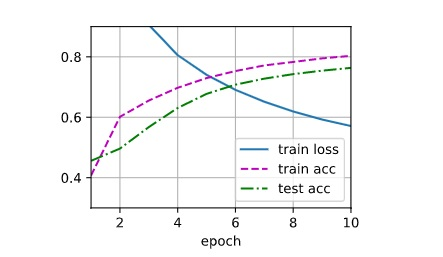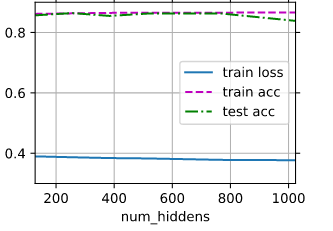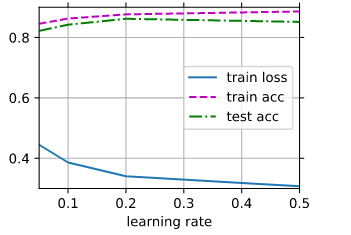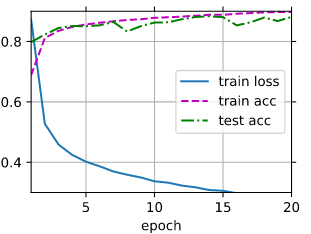# Implementation of Multilayer Perceptrons

I am getting lower train_acc/test_acc and higher train_loss (results attached as image).

Is there something wrong with my code ? My code is as follows:

from d2l import mxnet as d2l
from mxnet import gluon, np, npx, autograd
npx.set_np()
batch_size = 256

num_inputs, num_hidden, num_outputs = 784, 256, 10

w1 = np.random.normal(scale= 0.01,size = (num_inputs, num_hidden))
b1 = np.zeros(num_hidden)
w2 = np.random.normal(scale= 0.01, size = (num_hidden, num_outputs))
b2 = np.zeros(num_outputs)

params = [w1, b1, w2, b2]

for param in params:

def relu(X):
return np.maximum(X,0)

def net(X):
X = X.reshape((-1, num_inputs))
H = relu(np.dot(X,w1) + b1)
return np.dot(H,w2)+b2

loss = gluon.loss.SoftmaxCrossEntropyLoss()

num_epochs, lr = 10, 0.1

d2l.train_ch3(net, train_iter, test_iter, loss, num_epochs,
lambda batch_size: d2l.sgd(params, lr, batch_size) )

In here, we do not apply softmax function to the output layer, hence there may be some value which is negative and the sum of them not 1 (which should be to follow probabilty axiom). So how to decide the predicted class? the one with the maximam value? Am I missing something here about the explanation in why softmax is not applied? Thank you.

The reason that we don’t apply softmax in the implementation is that Cross Entropy Loss
takes care of the transformation. This is done to avoid any potential numeric overflow issues. If you look at the implementation of cross entropy loss in your preferred deep learning framework, you will find that there are several versions which take raw scores as inputs as it helps with the problems that are mentioned in http://d2l.ai/chapter_linear-networks/softmax-regression-concise.html. Hope this helps.

1 Like

Ah I see, it is about the engineering part and the framework has taken care of the caveat, thank you, now it’s crystal clear.

1 Like

task 1Best acc I got is batch_size is 1024
But test acc is not stable (

1. What is the smartest strategy you can think of for structuring a search over multiple hyper-
parameters?

-> Random search in the specified space for each parameter

Here is my findings for parameters:Looks like value near num_hidden=256 and lr=0.2 are optimal:So best accuracy reached on 15th epoch with near 90%